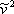# 9.1: Introduction

$$\newcommand{\vecs}{\overset { \rightharpoonup} {\mathbf{#1}} }$$ $$\newcommand{\vecd}{\overset{-\!-\!\rightharpoonup}{\vphantom{a}\smash {#1}}}$$$$\newcommand{\id}{\mathrm{id}}$$ $$\newcommand{\Span}{\mathrm{span}}$$ $$\newcommand{\kernel}{\mathrm{null}\,}$$ $$\newcommand{\range}{\mathrm{range}\,}$$ $$\newcommand{\RealPart}{\mathrm{Re}}$$ $$\newcommand{\ImaginaryPart}{\mathrm{Im}}$$ $$\newcommand{\Argument}{\mathrm{Arg}}$$ $$\newcommand{\norm}{\| #1 \|}$$ $$\newcommand{\inner}{\langle #1, #2 \rangle}$$ $$\newcommand{\Span}{\mathrm{span}}$$ $$\newcommand{\id}{\mathrm{id}}$$ $$\newcommand{\Span}{\mathrm{span}}$$ $$\newcommand{\kernel}{\mathrm{null}\,}$$ $$\newcommand{\range}{\mathrm{range}\,}$$ $$\newcommand{\RealPart}{\mathrm{Re}}$$ $$\newcommand{\ImaginaryPart}{\mathrm{Im}}$$ $$\newcommand{\Argument}{\mathrm{Arg}}$$ $$\newcommand{\norm}{\| #1 \|}$$ $$\newcommand{\inner}{\langle #1, #2 \rangle}$$ $$\newcommand{\Span}{\mathrm{span}}$$

If we multiply the vdW EOS (expression 7.11a) byand expand the factorized product by applying the distributive law, the result is the vdW EOS expressed in terms of molar volume, as follows:

$\bar{v}^{3}-\left(b+\frac{R T}{P}\right) \bar{v}^{2}+\left(\frac{a}{P}\right) \bar{v}-\frac{a b}{P}=0 \label{9.1}$

Note that Equation \ref{9.1} is a third order polynomial in $$\bar{v}$$ i.e., it is cubic in molar volume. Additionally, we can substitute the definition of compressibility factor $$Z$$,

$Z=\frac{P \bar{v}}{R T} \label{9.2}$

into Equation \ref{9.1} and obtain a different cubic polynomial in $$Z$$, as shown:

$Z^{3}-\left(1+\frac{b P}{R T}\right) Z^{2}+\left(\frac{a P}{R^{2} T^{2}}\right) Z-\frac{a b P^{2}}{(R T)^{3}}=0 \label{9.3}$

As we see, vdW EOS is referred to as cubic because it is a polynomial of order 3 in molar volume (and hence in compressibility factor $$Z$$). In general, any equation of state that is cubic in volume (and $$Z$$) and explicit in pressure (Equation 7.11b) is regarded as a cubic equation of state. vdW EOS is a cubic EOS, and all the transformations and modifications that it has undergone during the more than one hundred years since its publication are also cubic EOS; or better, they are in-the-van-der-Waals-spirit EOS or of-the-van-der-Waals-family EOS.